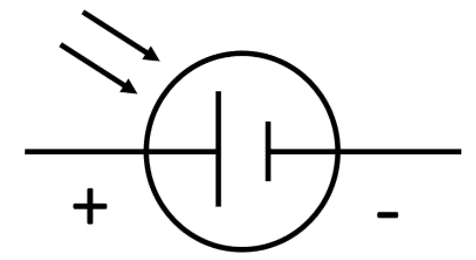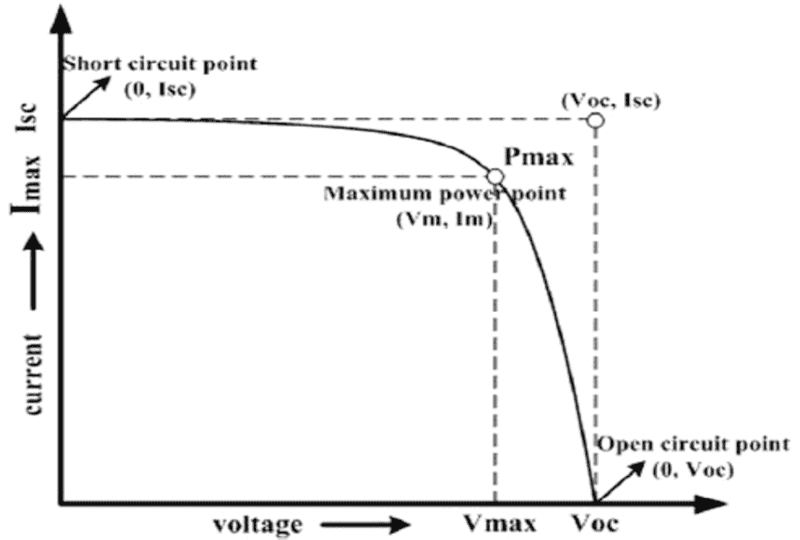# Connection of two photovoltaic cells to produce larger current

songoku
Homework Statement:
The symbol for photovoltaic cell is given below. The cell supplies current of magnitude ##I## at em.f. ##E##. Draw a diagram to show how two of these cells may be connected so that the combination supplies a total current greater than ##I## at the same e.m.f
Relevant Equations:
Series and ParallelThe answer from my teacher is two of these photovoltaic cells are connected in parallel. I can understand the parallel connection will produce same e.m.f but I don't understand why the total current will be greater than ##I##.

I imagined one of the cell connected to a lamp. The current through the lamp will be ##I##. And now there are two of these cells connected in parallel and I imagine a lamp also connected parallel to the two cells. The voltage on the lamp won't change so the current pssing thorugh it will also still be ##I##. What I think will change actually is the current provided by the cells, which is now becomes ##\frac I 2## but the total current would still be ##I##

Am I wrong? Thanks

Homework Helper
Gold Member
2022 Award
The voltage quoted for a solar cell is open circuit. As soon as it is connected up as a circuit and current flows the voltage falls. Having cells in parallel will reduce the decline.
Maximum power output is achieved by matching the load to the cell's effective internal resistance. See https://www.alternative-energy-tutorials.com/solar-power/measuring-the-power-of-a-solar-panel.html.
I agree that putting two in parallel will not in general double the total current, but if the load is doubled at the same time it will.

•songoku
songoku
The voltage quoted for a solar cell is open circuit. As soon as it is connected up as a circuit and current flows the voltage falls. Having cells in parallel will reduce the decline.
I understand this part. This is to fulfill the "at the same e.m.f" part.

I agree that putting two in parallel will not in general double the total current, but if the load is doubled at the same time it will.
But will putting two in parallel increase the total current to be larger than #I#?

And I don't understand why doubling the load will double the current. I thought since the value of e.m.f does not change (or just change a little bit due to potential drop in internal resistance), doubling the resistance will make the current becomes half of initial

Thanks

Homework Helper
Gold Member
2022 Award
I don't understand why doubling the load will double the current.
Sorry, I wrote that sloppily. I meant adding a second load in parallel.
If you take just the one cell and add a second load in parallel it won't double the current because as soon as the current starts to increase the voltage drop will too. But if you have two cells in parallel then, obviously, you can have a second equal load in parallel with no loss of voltage.

•songoku
Homework Helper
Gold Member
2022 Award
But will putting two in parallel increase the total current to be larger than #I#?
If you have one cell sending current through a load then the voltage will have dropped from the open circuit value. Adding a second cell in parallel will restore the voltage somewhat, leading to an increased current.

•songoku••